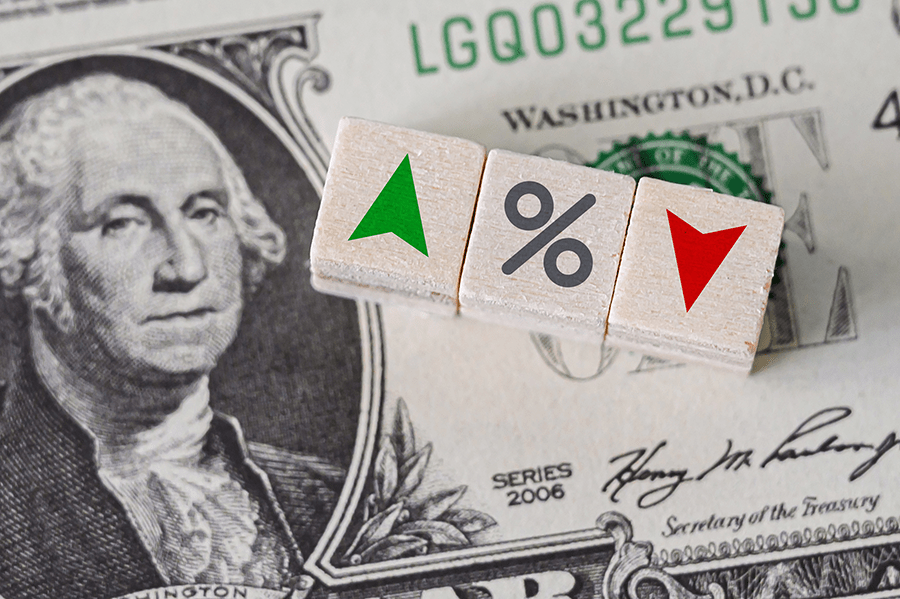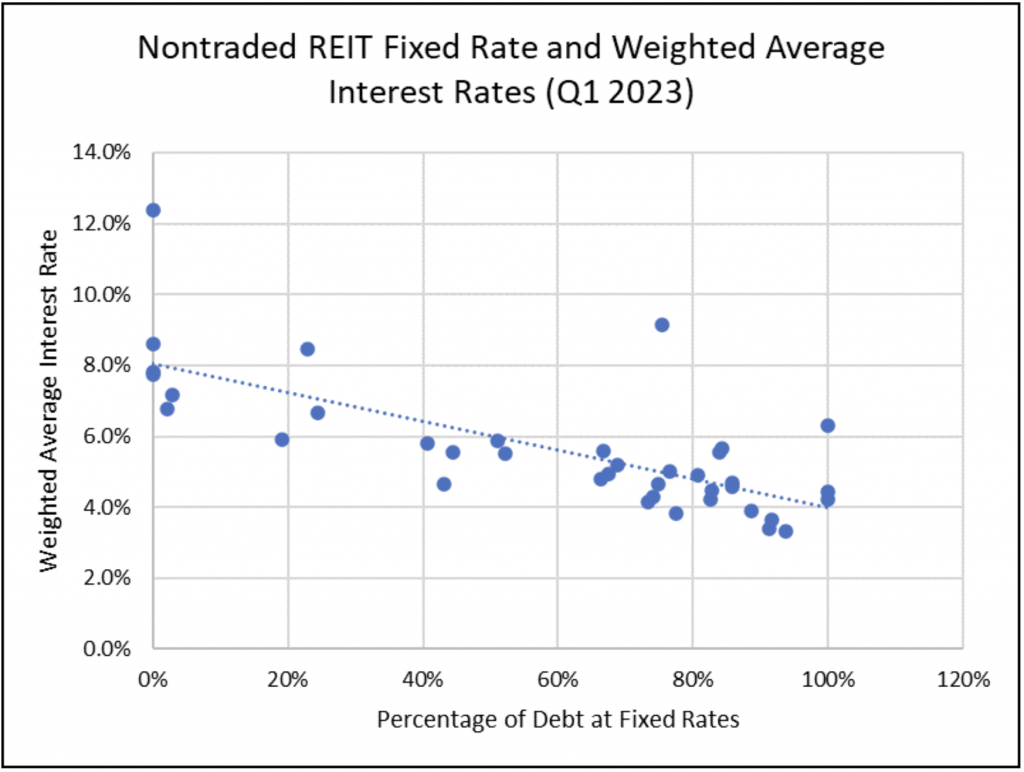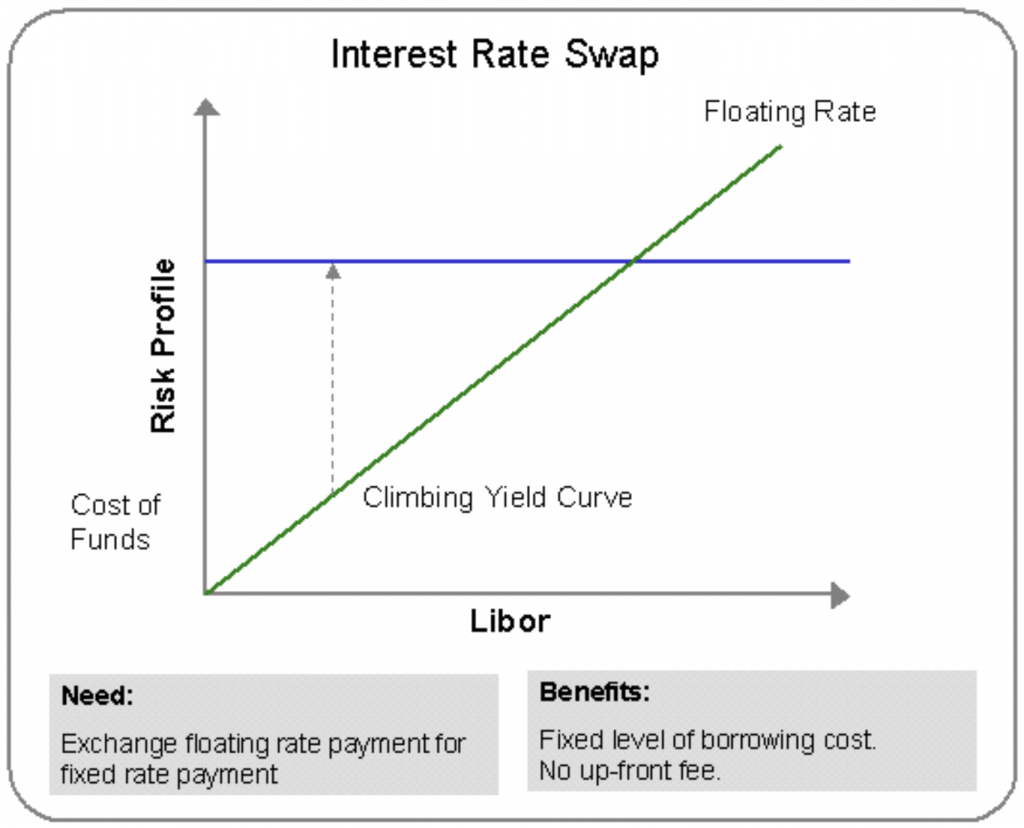August 29, 2023

# How Interest Rate Caps and Swaps Are Used by Non-Traded REITsAugust 29, 2023

# How Interest Rate Caps and Swaps Are Used by Non-Traded REITs

August 29, 2023 | James Sprow | Blue Vault

Non-traded REITs utilize different types of financial contracts to hedge their variable rate debts to protect themselves financially from changes in interest rates.  It is fair to say that the volatility of interest rates increases the risks to borrowers like non-traded REITs. Risk due to fluctuations in interest rates that make a REIT’s cash flows more variable are risks that investors need to be aware of, and mitigating those risks can create more certainty for investors and make investments in REITs more attractive.

With the rise in market interest rates since the spring of 2022, non-traded REITs have been using more hedging strategies to reduce the interest rate risks they face with their borrowings. There are two basic types of financial contracts that REITs use to mitigate interest rate risk related to their variable rate debt.  These are interest rate caps and swaps. Blue Vault’s analysts read the REIT filings to determine how much of the REIT’s debt has been effectively hedged, converting interest rates from variable rates to fixed rates.

An Example of the Impacts of Hedging Interest Rates

A simple illustration can show how potentially important converting variable rate debt to fixed rate debt can be. For example, Blackstone Real Estate Income Trust (“BREIT”) reported total indebtedness as of June 30, 2023, of \$68.1 billion. Of that debt, at least \$37.8 billion were reported as variable rate loans secured by the REIT’s properties. In the REIT’s Q2 2023, it reports a weighted average interest rate on loans secured by properties at 6.0%. The impact of interest rate swaps, caps and other derivatives reduced that weighted average interest rate by 1.7%, resulting in a net weighted average interest rate for those loans at approximately 4.3%1.  (The REIT also had hedging instruments with a notional value of \$0.9 billion related to their real estate debt investments that are at floating rates.)

How important is a 1.7% reduction in an effective interest rate in reducing interest rate expense? The reduction due to hedging contracts has the potential to reduce interest rate expense for BREIT by approximately \$1.16 billion per year. Interest expense for the six months ended June 30, 2023, totaled \$1.61 billion. Before considering the cost of purchasing hedging contracts, the reduction in interest expense could be roughly 26% of that for all 2023. [(1.16/ (2 x 1.61 + 1.16)) = 0.26)]

Looking at the financials filed by Blackstone REIT for Q2 2023, we do not find expenses recorded for hedging contracts such as interest rate caps and swaps. Rather, the 10-Q reports the net effects of the hedging contracts which could be the savings in interest expense less the cost of the swaps and caps.

How Are Nontraded REITs Moving Toward More Fixed Rate Debt?

It is intuitively clear that, in an era of rising market interest rates, nontraded REITs would be expected to hedge more of their debt. If they are successfully hedging and converting variable rate debt, there might be a relationship between the percentage of a REIT’s debt that is at fixed rates and the weighted average interest rate that the REIT is paying on their debt. Blue Vault reports both the weighted average interest rates and the percentage of fixed and variable rate debt for each nontraded REIT.

The chart below shows the relationship between the weighted average interest rate on the nontraded REITs’ debt and the percentage of the REITs’ debt at fixed rates. The chart reveals the relationship to be as we might expect. Certainly, other factors are involved, such as the size of the REIT in terms of AUM and the types of assets in the REIT portfolios. We expect larger REITs to have access to lower interest rates in their financing, and REITs with portfolios of multifamily assets to use more debt, other things equal. REITs that have succeeded in locking in lower interest rates by converting variable rate debt to fixed rate debt will tend to show up in the lower right of the chart. It appears that, of the 44 nontraded REITs for which we have this data as of Q1 2023, converting variable rate debt to fixed rate debt using hedging contracts in the current era of rising rates has apparently been successful.Understanding Interest Rate Swap Contracts

An interest rate swap is an agreement between two parties to exchange one stream of interest payments for another, over a set period. Swaps are derivative contracts and can be traded over-the-counter. In a swap contract, a nontraded REIT would exchange a variable rate of interest that applies to their borrowings for a fixed rate of interest. By purchasing an interest rate swap, the REIT commits to paying a fixed rate of interest instead of the variable rate for a loan used to finance its real estate acquisitions. The “counter-party” represents the position taken by another party to assume the responsibility to pay the variable rate of interest.

For example, if a property was financed with a \$100,000,000 mortgage at a variable rate of interest indexed to SOFR2 plus a spread, say the 30-day SOFR rate plus 1.50%, the REIT faces the risk of a rising reference rate increasing the interest owed on the debt. A swap contract could commit the firm to paying a fixed rate of 6.00% when the SOFR reference rate is at 3.50%, with the counter party assuming the risk of a rising SOFR. The chart below shows the possible effects of a swap.  Depending upon the agreed upon fixed rate, the swap may not require a fee. The swap can be valued at zero if the contract represents a “fair value” at the time it is purchased. That “fair value” of zero would be defined as the risk to either party to the swap being roughly equal.

The chart illustrates the cost of funds to the borrower at variable rates versus the cost when a swap is utilized (blue line).Swaps are often utilized if a company can borrow money more easily at one type of interest rate (variable) but prefers a different type (fixed). The lender is not directly involved in the swap.

Float-to-Float

Companies sometimes enter into a swap to change the type or tenor of the floating rate index that they pay; this is known as a basis swap. A company can swap from a three-month LIBOR to six-month LIBOR, for example, because the rate either is more attractive or matches other payment flows. A company can also switch to a different index, such as SOFR, the Federal Funds rate, commercial paper, or the Treasury bill rate.

Interest Rate Caps and Floors

An interest rate cap specifies that the seller agrees to pay the buyer if the reference rate exceeds the strike rate. An interest rate floor specifies that the seller agrees to pay the buyer if the reference rate is below the strike rate. Caps and floors can be thought of as option contracts. They have a strike rate, meaning the contract specifies when the floating or variable rate hits a certain point and triggers a conversion to a fixed rate for the owner of the cap. The seller of a cap takes on the risk of the variable rate exceeding the strike rate and does so for a fee or premium. By paying a fee or premium for the cap, the REIT must weigh the value of converting variable interest rates to fixed rates when the variable rates exceed the cap against the cost of the fee. A counter-party, such as a bank, can earn fees by entering into a cap contract with a REIT, and may structure its own risk profile by owning a portfolio of caps and swaps that effectively hedge its derivative portfolio’s interest rate risk.Blue Vault’s Nontraded REIT Reporting for Swaps and Caps

Our REIT analysts do their best to pull data from SEC filings by the REITs to determine how much of the REIT’s debt is effectively hedged. In doing so, we report the total of the REIT’s debt that remains variable and the portion that can be effectively considered at fixed rates. We do so each quarter, using the quarter’s end date in the calculation. We also give the REIT’s financial team the opportunity to respond to our preliminary draft, and we communicate with the REIT our definition of fixed and variable rate debt.

One principle we use in our reporting is, to be an effective hedge, a debt instrument that has a related interest cap contract must have a rate that has hit the strike rate. In other words, when the variable rate exceeds the strike, the interest rate on the debt becomes effectively fixed and can go no higher than the strike rate. A particular REIT might report that a debt’s interest rate is hedged because it has an interest rate cap, but until the variable or floating rate hits the cap’s strike rate, we consider it to be variable.

Footnotes

1. By reporting a weighted average rate, the REIT does not disclose how many of the debt instruments are subject to caps or swaps or the strike rates for those contracts. The reported interest rate adjustment may include the cost of hedging contracts, but that cost is not disclosed.
2. SOFR is the reference rate that became the approved reference rate of interest, replacing LIBOR. SOFR is the Secured Overnight Financing Rate. In 2022, the LIBOR Act passed by the U.S. Congress established SOFR as a default replacement rate for LIBOR contracts that lack mechanisms to deal with LIBOR’s cessation.